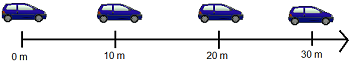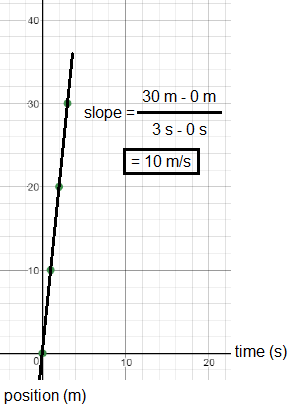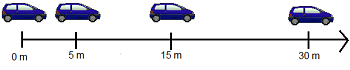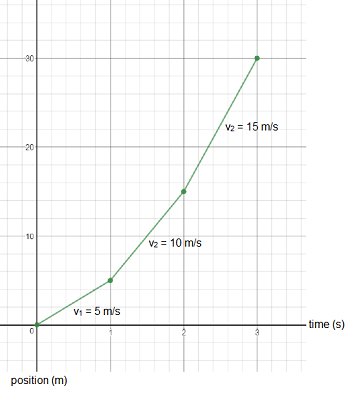# What Is Non-Uniform Motion? - Definition & Graph Analysis

An error occurred trying to load this video.

Try refreshing the page, or contact customer support.

Coming up next: Linear Momentum: Definition, Equation, and Examples

### You're on a roll. Keep up the good work!

Replay
Your next lesson will play in 10 seconds
• 0:01 What Is Uniform Motion?
• 1:00 What Is Non-Uniform Motion?
• 3:30 Lesson Summary

Want to watch this again later?

Timeline
Autoplay
Autoplay
Speed

#### Recommended Lessons and Courses for You

Lesson Transcript
Instructor: Betsy Chesnutt

Betsy teaches college physics, biology, and engineering and has a Ph.D. in Biomedical Engineering

Whenever a moving object changes its speed, we say that its motion is non-uniform. In this lesson, learn more about non-uniform motion and how to analyze it graphically.

## What Is Uniform Motion?

Imagine that you could take a picture of a car traveling down a straight road every second. It might look something like the diagram that you're seeing on your screen right now.Did you notice that the car travels the exact same distance every second? That's because this is an example of uniform motion, in which an object travels equal distances in equal time intervals. If any object has uniform motion, then it also has a constant velocity.

One way to represent the motion of an object is to make a position-time graph. If you made a graph of the car's position over the three seconds shown, it would look like the one on your screen right now.Looking at this graph, you can tell right away that the motion is uniform, because the graph forms a straight line with a constant slope. The slope of a position-time graph will tell you the velocity of an object, which is 10 m/s for the car shown earlier.

## What Is Non-Uniform Motion?

We've seen what uniform motion looks like, but objects don't always exhibit uniform motion. Many times, they speed up or slow down so that their motion is no longer uniform. Let's look at the car again, and see what's happening now.Does it still have uniform motion? No, you can see that now the car is going a different distance in each second. When objects like this car exhibit non-uniform motion, it means that they travel different distances in equal time intervals. In this case, during the first second the car travels 5 m. It travels 10 m during the next second, and then 15 m during the third second.

What would this look like on a position-time graph? Let's plot these points and see:Unlike the graph of uniform motion, this position-time graph of non-uniform motion does NOT have a constant slope, because the velocity of the car is changing every second. In the first second, the velocity is only 5 m/s, but this increases to 15 m/s by the third second.

When the velocity of a moving object changes, then it has an acceleration. Acceleration is defined as the rate of change of an object's velocity. To calculate acceleration graphically, we need a new type of graph. If you graph the object's velocity vs. time, the slope of the resulting velocity time graph will tell you how quickly the velocity of the object is changing, which is its acceleration.

To unlock this lesson you must be a Study.com Member.

### Register to view this lesson

Are you a student or a teacher?

### Unlock Your Education

#### See for yourself why 30 million people use Study.com

##### Become a Study.com member and start learning now.
Back
What teachers are saying about Study.com

### Earning College Credit

Did you know… We have over 160 college courses that prepare you to earn credit by exam that is accepted by over 1,500 colleges and universities. You can test out of the first two years of college and save thousands off your degree. Anyone can earn credit-by-exam regardless of age or education level.